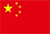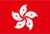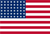Scanning QR Code• Online Inquiry

+
Congratulations
You've successfully got advanced quotes worth 5000 HKD
• Home
• Quotes
• News
• Community
• Learn
• Help Center
• Language
•••EN

# What Is The Price-to-earnings Ratio?

Views 32682022.05.19## Core points

The price-to-earnings ratio is calculated by dividing the share price by earnings per share.

The classification of P / E ratio mainly includes static P / E ratio, rolling P / E ratio and forecast P / E ratio.

The price-to-earnings ratio is mainly applied to the valuation of companies with stable profits.

## Detailed explanation of concept

The measure of whether a listed company is overvalued or undervalued is not by the absolute value of its share price, but by its relative value. The most common measure of relative valuation is the price / earnings ratio (PE).

The price-to-earnings ratio is calculated by dividing the share price by earnings per share and usually by the total market capitalization divided by net profit. Represents the increase in market value brought about by the net profit per unit of stock.

For example, if Tencent's market capitalization is HK \$4.6402 trillion and its 2020 profit is HK \$194.8 billion at the close of trading on November 10, 2021, Tencent's price-to-earnings ratio based on 2020 profits is 1,948 times earnings.

## Classification of price-earnings ratio

According to the time interval of selecting net profit data, the price-to-earnings ratio can be divided into the following types:

## Static price-earnings ratio

Based on the net profit of the past full fiscal year, the calculated price-to-earnings ratio is called static price-earnings ratio. The calculation of static P / E ratio is the simplest, but the disadvantage is that the valuation measurement lags behind.

## Rolling price-earnings ratio

Based on the rolling net profit of the past 12 months, the calculated price-to-earnings ratio is called the rolling price-earnings ratio. For example, after a listed company publishes its semi-annual report, the profit data selected by its latest rolling price-to-earnings ratio is the sum of the profits in the first half of this year and the second half of last year.

## Forecast price-earnings ratio

The calculated price-to-earnings ratio is called the forecast price-to-earnings ratio based on the forecast 12-month net profit. For example, after a listed company publishes its quarterly report, the annual forecast profit is obtained by multiplying the quarterly profit by 4, and then the forecast price-earnings ratio is calculated, which is a form of calculation of the forecast price-earnings ratio.

## Application of price-to-earnings ratio

The price-to-earnings ratio is usually used to measure the valuation of listed companies with stable profits, and for emerging companies with no profits or cyclical enterprises with unstable profits, similar valuation indicators such as price-to-sales ratio (market capitalization / revenue) and price-to-book ratio (market capitalization / net assets) are applied.

In addition, what investors value most is the sustainable profitability of listed companies. One-time gains and losses such as government subsidies or investment gains (losses) can be removed from the net profit, from which a more effective adjusted price-earnings ratio can be calculated.

Investors can compare the calculated price-earnings ratio of a company with the average price-earnings ratio of listed companies in the same industry, or with their own historical price-earnings ratio, to judge whether the valuation level of the company is reasonable.

Disclaimer: The above content does not constitute any act of financial product marketing, investment offer, or financial advice. Before making any investment decision, investors should consider the risk factors related to investment products based on their own circumstances and consult professional investment advisors where necessary.

### Recommended

Back to the Top